# 1 Introduction

The TreeSummarizedExperiment class is an extension of the SingleCellExperiment class (Lun and Risso 2019). It’s used to store rectangular data of experimental results as in a SingleCellExperiment, and also supports the storage of a hierarchical structure and its link information to the rectangular data.

# 2 TreeSummarizedExperiment

## 2.1 Anatomy of TreeSummarizedExperiment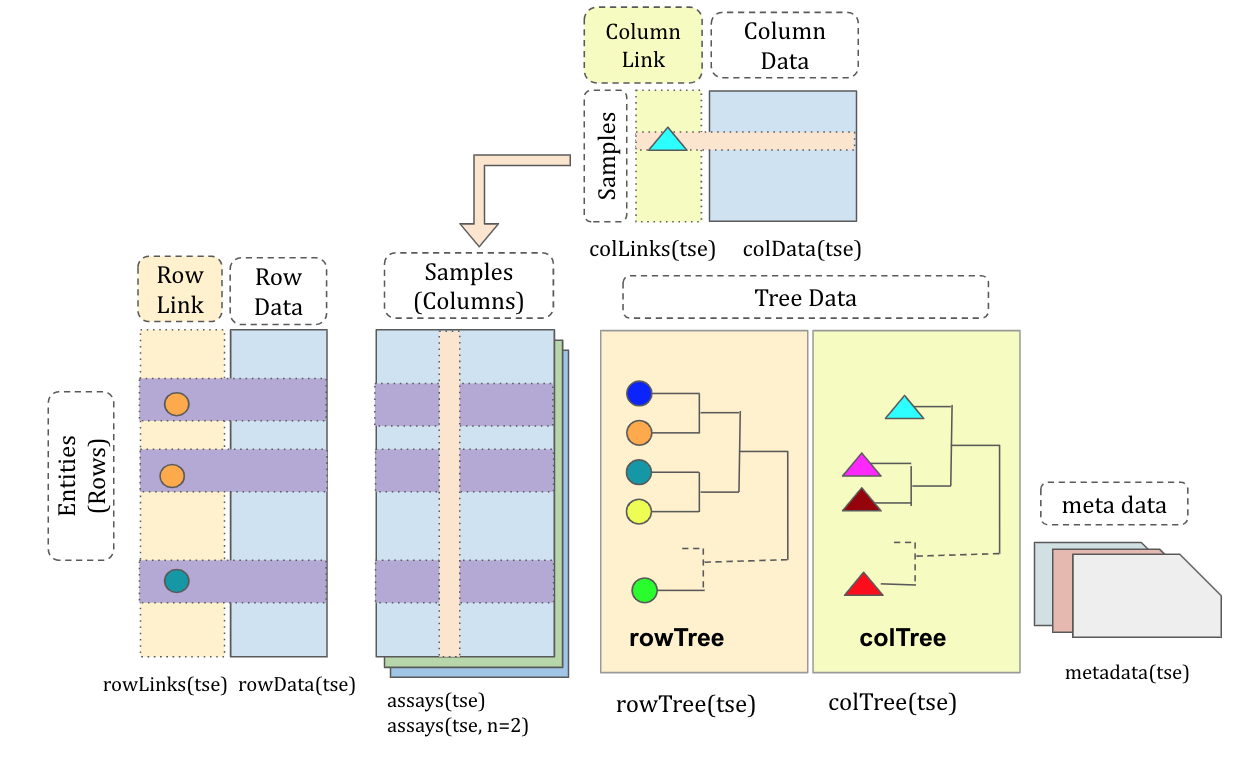Figure 1: The structure of the TreeSummarizedExperiment class

Compared with the SingleCellExperiment class, TreeSummarizedExperiment has four more slots.

• rowTree: the hierarchical structure on the rows of the assays tables.
• rowLinks: the link between rows of the assays tables and the rowTree.
• colTree: the hierarchical structure on the columns of the assays tables.
• colLinks: the link information between columns of assays tables and the colTree.

The rowTree and colTree could be empty (NULL) if no trees are available. Correspondingly, the rowLinks and colLinks would be NULL. All the other slots inTreeSummarizedExperimentare inherited fromSingleCellExperiment.

The slots rowTree and colTree only accept the tree data as the phylo class. If the tree is available in other formats, one would need to convert it to phylo with other R packages. For example, the package treeio provides 12 functions to import different tree formats and output phylo object in the slot phylo.

## 2.2 Toy data

suppressPackageStartupMessages({
library(TreeSummarizedExperiment)
library(S4Vectors)
library(ggtree)
library(ape)})

We generate a assay_data with observations of 5 entities collected from 4 samples.

# assays data
assay_data <- rbind(rep(0, 4), matrix(1:16, nrow = 4))
colnames(assay_data) <- paste(rep(LETTERS[1:2], each = 2),
rep(1:2, 2), sep = "_")
rownames(assay_data) <- paste("entity", seq_len(5), sep = "")
assay_data
##         A_1 A_2 B_1 B_2
## entity1   0   0   0   0
## entity2   1   5   9  13
## entity3   2   6  10  14
## entity4   3   7  11  15
## entity5   4   8  12  16

The descriptions of the 5 entities and 4 samples are given in the row_data and col_data, respectively.

# row data
row_data <- DataFrame(var1 = sample(letters[1:2], 5, replace = TRUE),
var2 = sample(c(TRUE, FALSE), 5, replace = TRUE),
row.names = rownames(assay_data))
row_data
## DataFrame with 5 rows and 2 columns
##                var1      var2
##         <character> <logical>
## entity1           b      TRUE
## entity2           a     FALSE
## entity3           a      TRUE
## entity4           a      TRUE
## entity5           b     FALSE
# column data
col_data <- DataFrame(gg = c(1, 2, 3, 3),
group = rep(LETTERS[1:2], each = 2),
row.names = colnames(assay_data))
col_data
## DataFrame with 4 rows and 2 columns
##            gg       group
##     <numeric> <character>
## A_1         1           A
## A_2         2           A
## B_1         3           B
## B_2         3           B

The hierarchical structure of the 5 entities is denoted as row_tree. The hierarchical structure of the 4 samples is denoted as col_tree. We create them by using the function rtree from the package ape.

# Toy tree 1
set.seed(1)
row_tree <- rtree(5)
class(row_tree)
##  "phylo"
# Toy tree 2
set.seed(4)
col_tree <- rtree(4)
col_tree$tip.label <- colnames(assay_data) col_tree$node.label <- c("All", "GroupA", "GroupB")

The created trees are phylo objects. The phylo object is actually a list with at least four elements: edge, tip.label, edge.length, and Nnode.

class(row_tree)
##  "phylo"
str(row_tree)
## List of 4
##  $edge : int [1:8, 1:2] 6 6 7 8 8 9 9 7 1 7 ... ##$ tip.label  : chr [1:5] "t2" "t1" "t3" "t4" ...
##  $edge.length: num [1:8] 0.0618 0.206 0.1766 0.687 0.3841 ... ##$ Nnode      : int 4
##  - attr(*, "class")= chr "phylo"
##  - attr(*, "order")= chr "cladewise"

The package ggtree (Yu et al. 2017) has been used to visualize the tree. The node labels and node numbers are in blue and orange texts, respectively. The row_tree has no labels for internal nodes.

# Visualize the row tree
ggtree(row_tree, size = 2) +
geom_text2(aes(label = node), color = "darkblue",
hjust = -0.5, vjust = 0.7, size = 6) +
geom_text2(aes(label = label), color = "darkorange",
hjust = -0.1, vjust = -0.7, size = 6)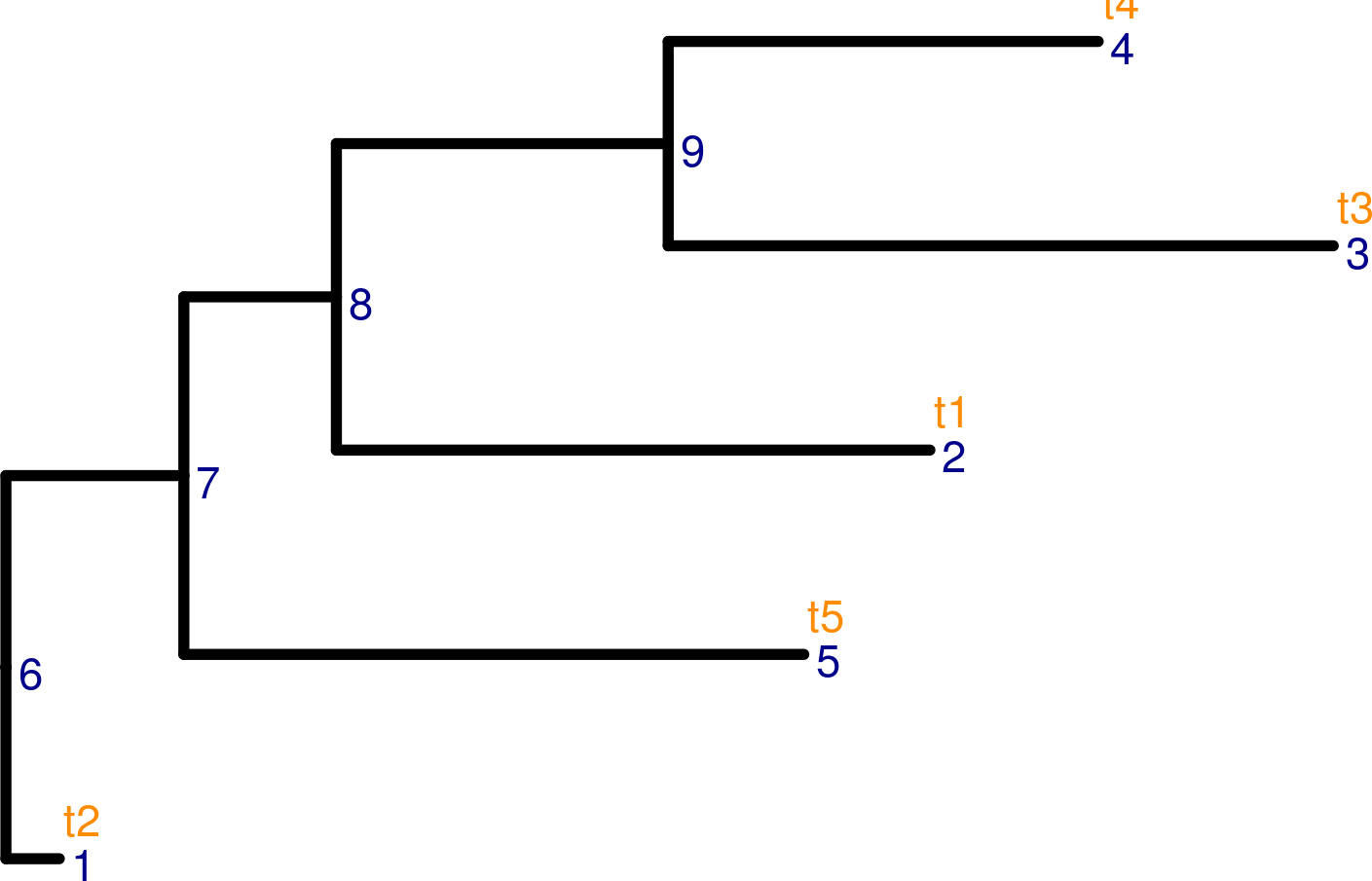Figure 2: The structure of the row tree

The col_tree has labels for internal nodes.

# Visualize the column tree
ggtree(col_tree, size = 2) +
geom_text2(aes(label = node), color = "darkblue",
hjust = -0.5, vjust = 0.7, size = 6) +
geom_text2(aes(label = label), color = "darkorange",
hjust = -0.1, vjust = -0.7, size = 6)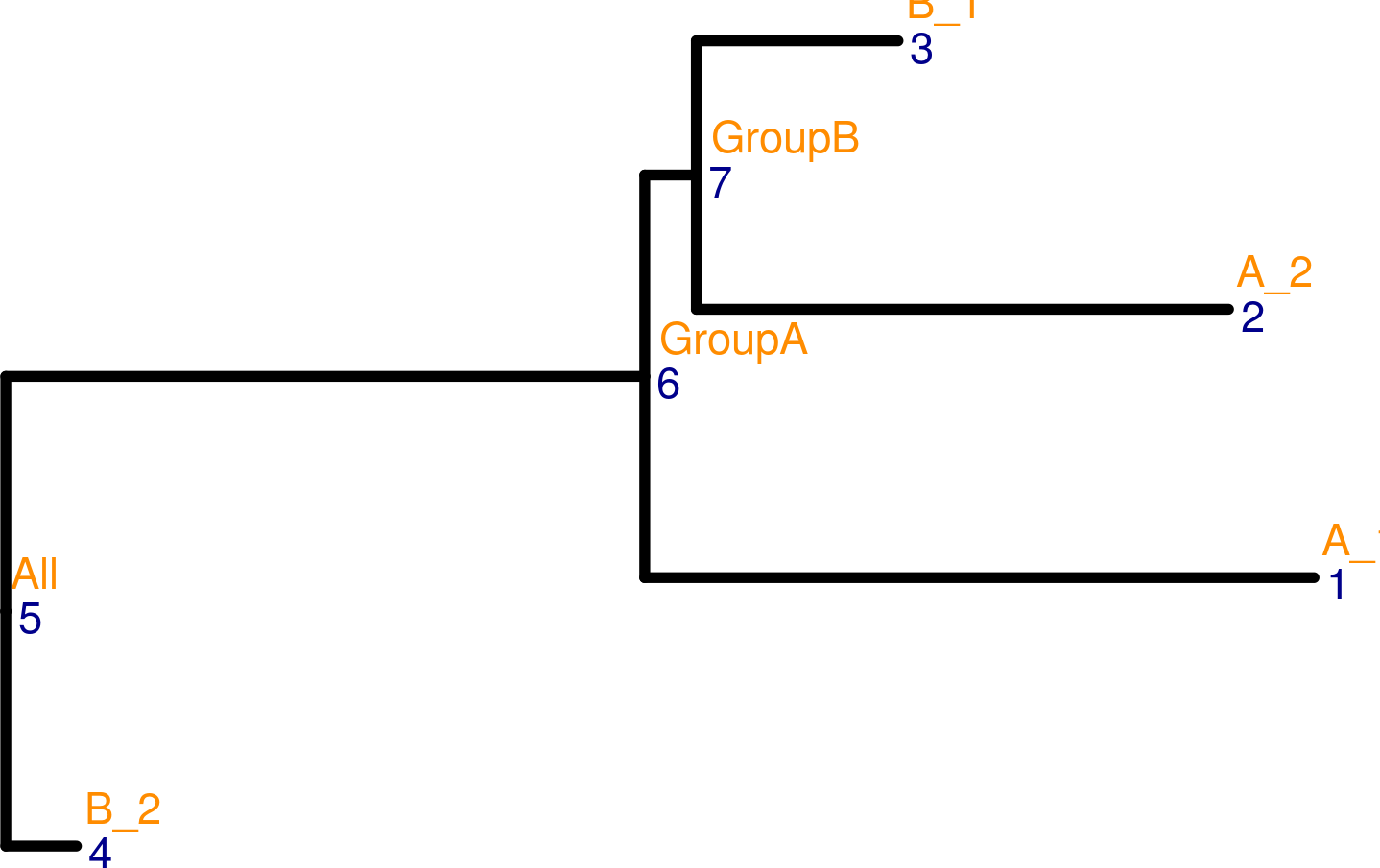Figure 3: The structure of the column tree

## 2.3 The construction of TreeSummarizedExperiment

The TreeSummarizedExperiment class is used to store the toy data: assay_data, row_data, col_data, col_tree and row_tree, To correctly store data, the link information between the rows (or columns) of assay_data and the nodes of the row_tree (or col_tree) is requried to provide via a charactor vector rowNodeLab (or colNodeLab). Those columns or rows that don’t match with any node of the tree structure are removed with warnings. The link data between the assays tables and the tree data is automatically generated in the construction.

Below shows an example to construct TreeSummarizedExperiment without the column tree.

# provide the node labels in rowNodeLab
node_lab <- row_tree$tip.label row_tse <- TreeSummarizedExperiment(assays = list(assay_data), rowData = row_data, colData = col_data, rowTree = row_tree, rowNodeLab = node_lab) When printing out row_tse, we see a similar message as SingleCellExperiment with four additional lines about rowLinks, rowTree, colLinks and colTree. Here, row_tse stores a row tree (phylo object), and the rowLinks has 5 rows that is exactly the same as the number of rows in the assays tables. More details about the link data could be found in Section 2.4.2. row_tse ## class: TreeSummarizedExperiment ## dim: 5 4 ## metadata(0): ## assays(1): '' ## rownames(5): entity1 entity2 entity3 entity4 entity5 ## rowData names(2): var1 var2 ## colnames(4): A_1 A_2 B_1 B_2 ## colData names(2): gg group ## reducedDimNames(0): ## spikeNames(0): ## altExpNames(0): ## rowLinks: a LinkDataFrame (5 rows) ## rowTree: a phylo (5 leaves) ## colLinks: NULL ## colTree: NULL If the row tree and the column tree are both available, the TreeSummarizedExperiment could be constructed similarly as below. Here, the column names of the assays table match with the node labels used in the column tree. So, we could omit the step of providing colNodeLab. all(colnames(assay_data) %in% c(col_tree$tip.label, col_tree$node.label)) ##  TRUE both_tse <- TreeSummarizedExperiment(assays = list(assay_data), rowData = row_data, colData = col_data, rowTree = row_tree, rowNodeLab = node_lab, colTree = col_tree) Compared to row_tse, both_tse includes also a column tree. The column link data (colLinks) with 4 rows is automatically generated. The number of rows in the link data is decided by the column dimension of the assays tables. both_tse ## class: TreeSummarizedExperiment ## dim: 5 4 ## metadata(0): ## assays(1): '' ## rownames(5): entity1 entity2 entity3 entity4 entity5 ## rowData names(2): var1 var2 ## colnames(4): A_1 A_2 B_1 B_2 ## colData names(2): gg group ## reducedDimNames(0): ## spikeNames(0): ## altExpNames(0): ## rowLinks: a LinkDataFrame (5 rows) ## rowTree: a phylo (5 leaves) ## colLinks: a LinkDataFrame (4 rows) ## colTree: a phylo (4 leaves) ## 2.4 The accessor functions ### 2.4.1 Assays, rowData, colData, and metadata For slots inherited from the SingleCellExperiment class, the accessors are exactly the same as shown in SingleCellExperiment. # to get the first table in the assays (count <- assays(both_tse)[]) ## A_1 A_2 B_1 B_2 ## entity1 0 0 0 0 ## entity2 1 5 9 13 ## entity3 2 6 10 14 ## entity4 3 7 11 15 ## entity5 4 8 12 16 # to get row data rowData(both_tse) ## DataFrame with 5 rows and 2 columns ## var1 var2 ## <character> <logical> ## entity1 b TRUE ## entity2 a FALSE ## entity3 a TRUE ## entity4 a TRUE ## entity5 b FALSE # to get column data colData(both_tse) ## DataFrame with 4 rows and 2 columns ## gg group ## <numeric> <character> ## A_1 1 A ## A_2 2 A ## B_1 3 B ## B_2 3 B # to get metadata: it's empty here metadata(both_tse) ## list() ### 2.4.2 rowLinks, colLinks The row link and column link could be accessed via rowLinks and colLinks, respectively. The output would be a LinkDataFrame object. The LinkDataFrame class is extended from the DataFrame class with the restriction that it has at least four columns: nodeLab, nodeLab_alias, nodeNum, and isLeaf. More details about the DataFrame class could be found in the S4Vectors package. • nodeLab: the labels of nodes on the tree • nodeLab_alias: the alias labels of nodes on the tree • nodeNum: the numbers of nodes on the tree • isLeaf: it’s to indicate whether the node is a leaf node When a phylo tree is available in the rowTree, we could see a LinkDataFrame object in the rowLinks. The number of rows of rowLinks data matches with the number of rows of assays tables. (rLink <- rowLinks(both_tse)) ## LinkDataFrame with 5 rows and 4 columns ## nodeLab nodeLab_alias nodeNum isLeaf ## <character> <character> <integer> <logical> ## 1 t2 alias_1 1 TRUE ## 2 t1 alias_2 2 TRUE ## 3 t3 alias_3 3 TRUE ## 4 t4 alias_4 4 TRUE ## 5 t5 alias_5 5 TRUE class(rLink) ##  "LinkDataFrame" ## attr(,"package") ##  "TreeSummarizedExperiment" showClass("LinkDataFrame") ## Class "LinkDataFrame" [package "TreeSummarizedExperiment"] ## ## Slots: ## ## Name: rownames nrows listData ## Class: character_OR_NULL integer list ## ## Name: elementType elementMetadata metadata ## Class: character DataTable_OR_NULL list ## ## Extends: ## Class "DFrame", directly ## Class "LinkDataFrame_Or_NULL", directly ## Class "DataFrame", by class "DFrame", distance 2 ## Class "DataTable", by class "DFrame", distance 3 ## Class "SimpleList", by class "DFrame", distance 3 ## Class "DataTable_OR_NULL", by class "DFrame", distance 4 ## Class "List", by class "DFrame", distance 4 ## Class "Vector", by class "DFrame", distance 5 ## Class "list_OR_List", by class "DFrame", distance 5 ## Class "Annotated", by class "DFrame", distance 6 ## Class "vector_OR_Vector", by class "DFrame", distance 6 nrow(rLink) == nrow(both_tse) ##  TRUE Similarly, the number of rows of colLinks data matches with the number of columns of assays table. (cLink <- colLinks(both_tse)) ## LinkDataFrame with 4 rows and 4 columns ## nodeLab nodeLab_alias nodeNum isLeaf ## <character> <character> <integer> <logical> ## 1 A_1 alias_1 1 TRUE ## 2 A_2 alias_2 2 TRUE ## 3 B_1 alias_3 3 TRUE ## 4 B_2 alias_4 4 TRUE nrow(cLink) == ncol(both_tse) ##  TRUE If the tree is not available, the corresponding link data is NULL. colTree(row_tse) ## NULL colLinks(row_tse) ## NULL The link data is automatically generated when constructing the TreeSummarizedExperiment object. We highly recommend users not to modify it manually; otherwise the link might be broken. For R packages developers, we show in the Section 5.2 about how to update the link. ## 2.5 The subseting function We could use [ to subset the TreeSummarizedExperiment. To keep track of the original data, the rowTree and colTree stay the same in the subsetting. sub_tse <- both_tse[1:2, 1] sub_tse ## class: TreeSummarizedExperiment ## dim: 2 1 ## metadata(0): ## assays(1): '' ## rownames(2): entity1 entity2 ## rowData names(2): var1 var2 ## colnames(1): A_1 ## colData names(2): gg group ## reducedDimNames(0): ## spikeNames(0): ## altExpNames(0): ## rowLinks: a LinkDataFrame (2 rows) ## rowTree: a phylo (5 leaves) ## colLinks: a LinkDataFrame (1 rows) ## colTree: a phylo (4 leaves) The annotation data on the row and column dimension is changed accordingly. # The first four columns are from rowLinks data and the others from rowData cbind(rowLinks(sub_tse), rowData(sub_tse)) ## DataFrame with 2 rows and 6 columns ## nodeLab nodeLab_alias nodeNum isLeaf var1 var2 ## <character> <character> <integer> <logical> <character> <logical> ## 1 t2 alias_1 1 TRUE b TRUE ## 2 t1 alias_2 2 TRUE a FALSE # The first four columns are from colLinks data and the others from colData cbind(colLinks(sub_tse), colData(sub_tse)) ## DataFrame with 1 row and 6 columns ## nodeLab nodeLab_alias nodeNum isLeaf gg group ## <character> <character> <integer> <logical> <numeric> <character> ## 1 A_1 alias_1 1 TRUE 1 A # 3 Aggregation The aggregation is allowed on the row and the column dimension. ## 3.1 The column dimension Here, we show the aggregation on the column dimension. The TreeSummarizedExperiment object is assigned to the argument x. The desired aggregation level is given in colLevel. The level could be specified via the node label (the orange texts in Figure 3) or the node number (the blue texts in Figure 3). We could further decide how to aggregate via the argument FUN. # use node labels to specify colLevel aggCol <- aggValue(x = both_tse, colLevel = c("GroupA", "GroupB"), FUN = sum) # or use node numbers to specify colLevel aggCol <- aggValue(x = both_tse, colLevel = c(6, 7), FUN = sum) assays(aggCol)[] ## alias_6 alias_7 ## entity1 0 0 ## entity2 15 14 ## entity3 18 16 ## entity4 21 18 ## entity5 24 20 The rowData doesn’t change, but the colData adjusts with the change of the table. For example, the column group has the A value for GroupA because the descendant nodes of GroupA all have the value A; the column gg has the NA value for GroupA because the descendant nodes of GroupA have different values, (1 and 2). # before aggregation colData(both_tse) ## DataFrame with 4 rows and 2 columns ## gg group ## <numeric> <character> ## A_1 1 A ## A_2 2 A ## B_1 3 B ## B_2 3 B # after aggregation colData(aggCol) ## DataFrame with 2 rows and 2 columns ## gg group ## <logical> <logical> ## alias_6 NA NA ## alias_7 NA NA The colLinks is updated to link the new rows of assays tables and the column tree. # the link data is updated colLinks(aggCol) ## LinkDataFrame with 2 rows and 4 columns ## nodeLab nodeLab_alias nodeNum isLeaf ## <character> <character> <integer> <logical> ## 1 GroupA alias_6 6 FALSE ## 2 GroupB alias_7 7 FALSE From the Figure 2, we could see that the nodes 6 and 7 are labelled with GroupA and GroupB, respectively. This agrees with the column link data. ## 3.2 The row dimension It’s similar to the aggregation on the row dimension, except that the level should be specified via rowLevel. agg_row <- aggValue(x = both_tse, rowLevel = 7:9, FUN = sum) Now, the output assays table has 3 rows. assays(agg_row)[] ## A_1 A_2 B_1 B_2 ## alias_7 10 26 42 58 ## alias_8 6 18 30 42 ## alias_9 5 13 21 29 We could see which row corresponds to which nodes via the rowLinks data. rowLinks(agg_row) ## LinkDataFrame with 3 rows and 4 columns ## nodeLab nodeLab_alias nodeNum isLeaf ## <character> <character> <integer> <logical> ## 1 NA alias_7 7 FALSE ## 2 NA alias_8 8 FALSE ## 3 NA alias_9 9 FALSE The Figure 2 shows that the nodes 7, 8 and 9 have no labels. Therefore, the nodeLab column in LinkData of the row data has missing value. They are all internal nodes and hence the column isLeaf has only FALSE value. ## 3.3 Both dimensions The aggregation on both row and column dimensions could be performed in one step using the same function specified via FUN. If different functions are required for different dimension, it’s suggested to do it in two steps as described in Section 3.2 and Section 3.1 because the order of aggregation might matter. agg_both <- aggValue(x = both_tse, colLevel = c(6, 7), rowLevel = 7:9, FUN = sum) As expected, we obtain a table with 3 rows (rowLevel = 7:9) and 2 columns (colLevel = c(6, 7)). assays(agg_both)[] ## alias_6 alias_7 ## alias_7 78 68 ## alias_8 54 48 ## alias_9 39 34 # 4 Aggregation on the taxonomic table In some case, the information of the hierarchical structure is available as a data.frame instead of the phylo object mentioned above. To do the work listed above, we could convert the data.frame to the phylo class. The function toTree outputs the hierarchical information into a phylo object. If the data set is large, we suggest to allow cache = TRUE to speed up the aggregation step. # The toy taxonomic table taxa <- data.frame(Kindom = rep("A", 5), Phylum = c("B1", rep("B2", 4)), Class = c("C1", "C2", "C3", "C3", NA), OTU = c("D1", "D2", "D3", "D4", NA)) # convert to a phylo tree taxa_tree <- toTree(data = taxa, cache = FALSE) ggtree(taxa_tree)+ geom_text2(aes(label = node), color = "darkblue", hjust = -0.5, vjust = 0.7, size = 6) + geom_text2(aes(label = label), color = "darkorange", hjust = -0.1, vjust = -0.7, size = 6) + geom_point2()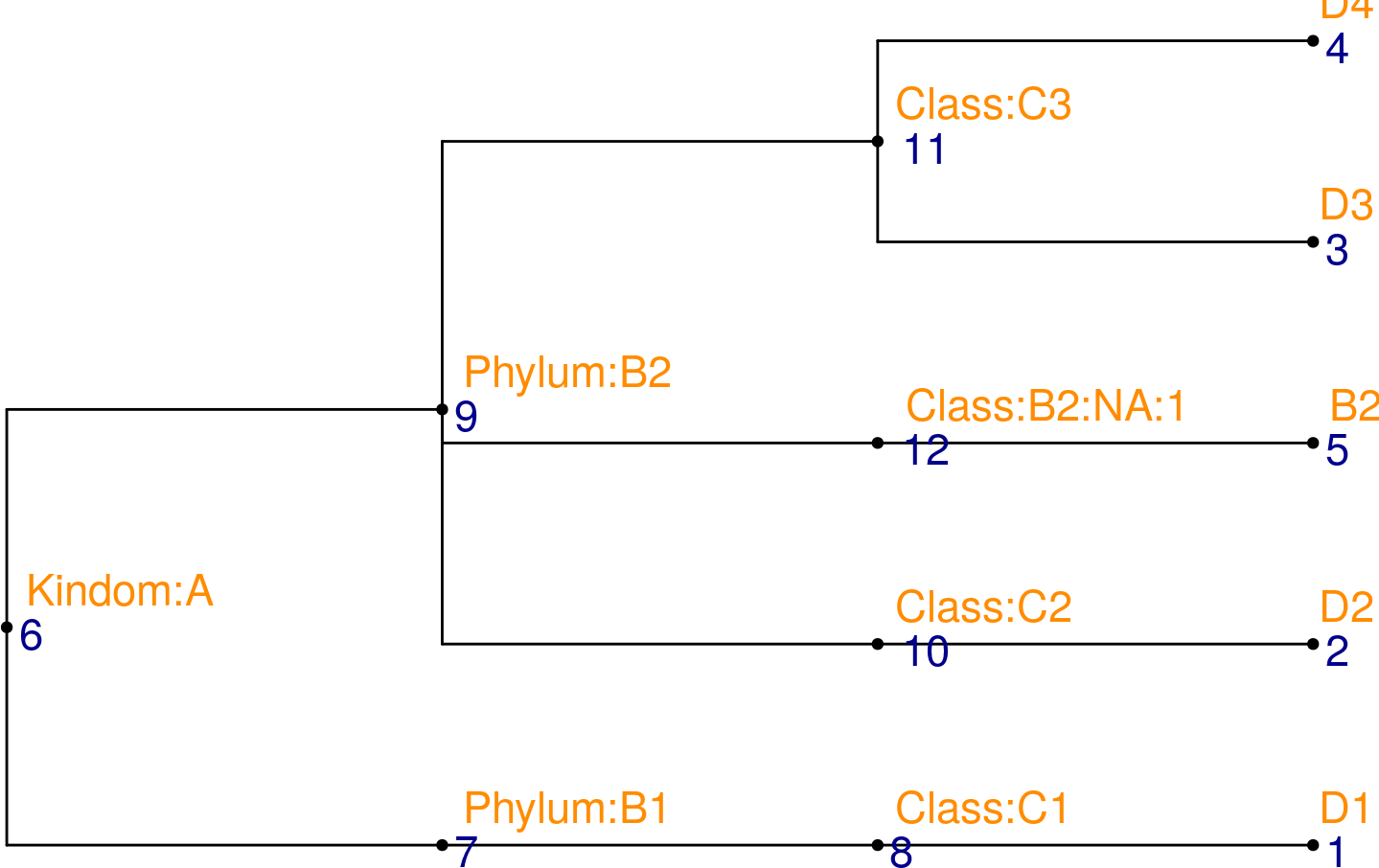# construct a TreeSummarizedExperiment object taxa_tse <- TreeSummarizedExperiment(assays = list(assay_data), rowData = row_data, rowTree = taxa_tree, rowNodeLab = taxa_tree$tip.label)

Here is about how to aggregate to the phylum level.

# specify the level
taxa_lab <- c(taxa_tree$tip.label, taxa_tree$node.label)
ii <- startsWith(taxa_lab, "Phylum:")
(l1 <- taxa_lab[ii])
##  "Phylum:B1" "Phylum:B2"
# aggregate
agg_taxa <- aggValue(x = taxa_tse, rowLevel = l1, FUN = sum)
assays(agg_taxa)[]
##         A_1 A_2 B_1 B_2
## alias_7   0   0   0   0
## alias_9  10  26  42  58
rowData(agg_taxa)
## DataFrame with 2 rows and 2 columns
##                var1      var2
##         <character> <logical>
## alias_7           b      TRUE
## alias_9          NA        NA

The aggregation could be on any freely combined level.

# specify the level
l2 <- c("Class:C3", "Phylum:B1")
# aggregate
agg_any <- aggValue(x = taxa_tse, rowLevel = l2, FUN = sum)
assays(agg_any)[]
##          A_1 A_2 B_1 B_2
## alias_11   5  13  21  29
## alias_7    0   0   0   0
rowData(agg_any)
## DataFrame with 2 rows and 2 columns
##                 var1      var2
##          <character> <logical>
## alias_11           a      TRUE
## alias_7            b      TRUE

## 5.1 More about functions working on the phylo object.

Here, we show some functions as examples to manipulate or to extract information from the phylo object. More functions could be found in other packages, such as ape (Paradis and Schliep 2018), tidytree. These functions might be useful when R package developers want to create their own functions to work on the TreeSummarizedExperiment class.

Below shows the node label (black texts) and node number (blue texts) of each node on an example tree.

ggtree(tinyTree, branch.length = "none") +
geom_text2(aes(label = label), hjust = -0.3) +
geom_text2(aes(label = node), vjust = -0.8,
hjust = -0.3, color = 'blue')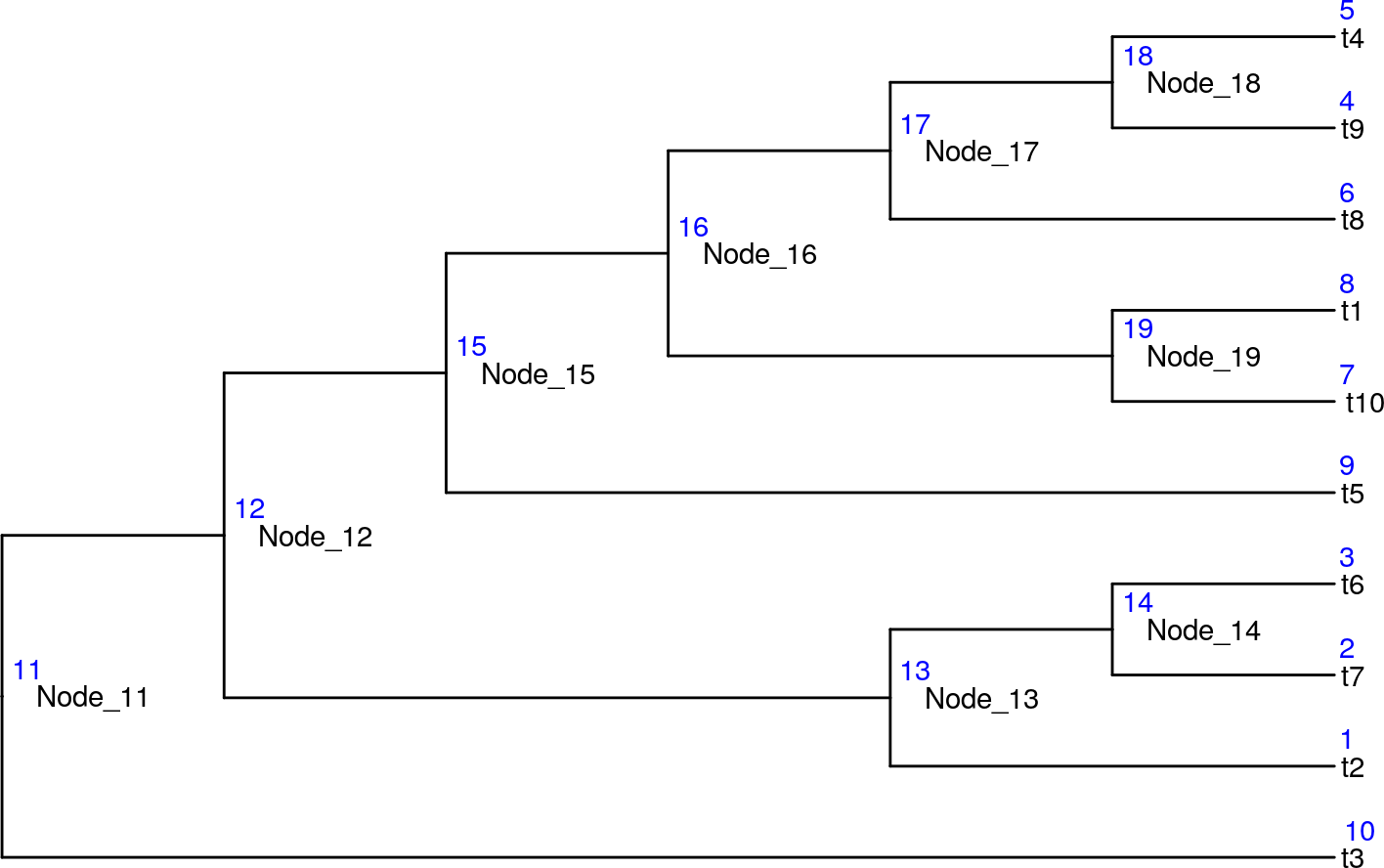### 5.1.2 Count the number of nodes

# The number of leaves
countLeaf(tree = tinyTree)
##  10
# The number of nodes (leaf nodes and internal nodes)
countNode(tree = tinyTree)
##  19

### 5.1.3 Translation between the node label and the node number

The translation between the labels and the numbers of nodes could be achieved by the function transNode.

transNode(tree = tinyTree, node = c(12, 1, 4))
##  "Node_12" "t2"      "t9"
transNode(tree = tinyTree, node = c("t4", "Node_18"))
##      t4 Node_18
##       5      18

### 5.1.4 find the descendants

To get descendants that are on the leaf level, we could set the argument only.leaf = `